0
0
01. 云栖社区>
2. 博客>
3. 正文

剑指offer第二章——c++实现 持续更新中

2.1面试官谈基础知识

1、c++的基础知识（面向对象的特性、构造函数、析构函数、动态绑定、内存管理）

2、设计模式

3、uml图

4、并发控制

5、对os的理解程度

2.2 编程语言

c++三种考查方式：

1、考概念（重点考察c++关键字的理解程度。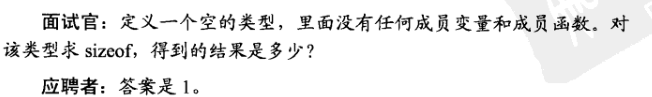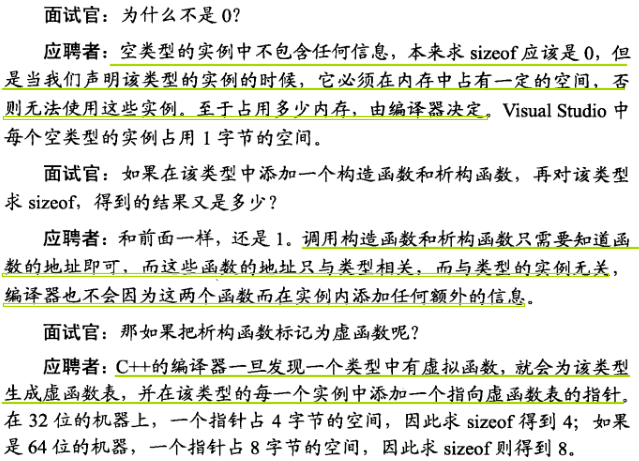2、分析代码的运行结果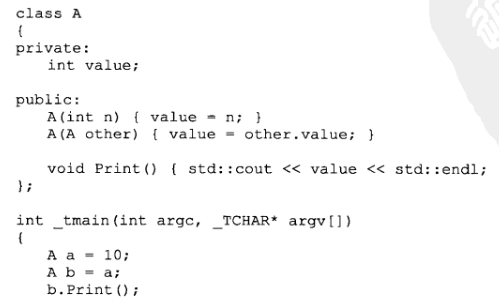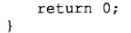A b = a;

（1）显式或隐式地用同类型的一个对象来初始化另外一个对象。如上例中的 A b = a;

（2）作为实参传递给一个函数。如上例中的bbb.myTestFunc(aaa);

（3）在函数体内返回一个对象时，也会调用返回值类型的拷贝构造函数

1)． 一个对象以值传递的方式传入函数体

2)． 一个对象以值传递的方式从函数返回

3)． 一个对象需要通过另外一个对象进行初始化

面试题一   考点：拷贝赋值函数的书写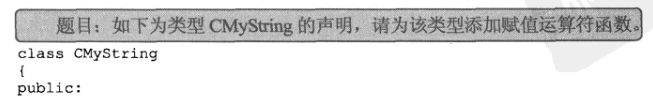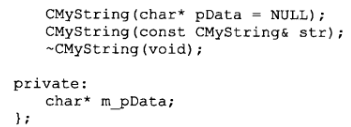1、是否把返回值的类型声明为该类型的引用，并在函数结束前返回实例自身的引用（*this）。只有返回一个引用，才可以运行连续赋值

2、是否把传入的参数类型声明为常量引用。如果传入的参数不是引用而是实例，那么从形参到实参会调用一次拷贝构造函数，把参数声明为引用可以避免这样的无谓消耗。

3、是否释放实例自身已有的内存（不释放会发生内存泄漏）

4、判断传入的参数和当前实例（*this）是否为同一个实例。如果是，则不进行复制操作，直接返回。

#pragma once
#include <cstring>
class CMyString {
public:
CMyString(char * pData = nullptr);
CMyString(const CMyString& STR);
CMyString& operator= (const CMyString& STR);
~CMyString(void);
private:
char * m_pData;
};

inline
CMyString& CMyString::operator=(const CMyString& str) {
if (this == &str)
return *this;

delete[] m_pData;
m_pData = new char[strlen(str.m_pData) + 1];
strcpy(m_pData, str.m_pData);
return *this;
}

1、先用new分配内存，在delete原来的内容。

2、先创建一个临时实例，在交换临时实例和原来的实例。（推荐）

#pragma once
#include <cstring>
class CMyString {
public:
CMyString(char * pData = nullptr);
CMyString(const CMyString& STR);
CMyString& operator= (const CMyString& STR);
~CMyString(void);
private:
char * m_pData;
};

inline
CMyString& CMyString::operator=(const CMyString& str) {
if (this != &str) {
CMyString strTemp(str);

char * pTemp = strTemp.m_pData;
m_pData = pTemp;
}
return *this;
}

#ifndef __MYSTRING__
#define __MYSTRING__

class String
{
public:
String(const char* cstr=0);
String(const String& str);
String& operator=(const String& str);
~String();
char* get_c_str() const { return m_data; }
private:
char* m_data;
};

#include <cstring>

//构造函数
inline
String::String(const char* cstr)
{
if (cstr) {
m_data = new char[strlen(cstr)+1];
strcpy(m_data, cstr);
}
else {
m_data = new char;
*m_data = '\0';
}
}

//拷贝构造
inline
String::String(const String& str)
{
m_data = new char[strlen(str.m_data) + 1];
strcpy(m_data, str.m_data);
}

//拷贝赋值
inline
String& String::operator=(const String& str)
{
if (this == &str)
return *this;

delete[] m_data;
m_data = new char[ strlen(str.m_data) + 1 ];
strcpy(m_data, str.m_data);
return *this;
}

//析构函数
inline
String::~String()
{
delete[] m_data;
}

#endif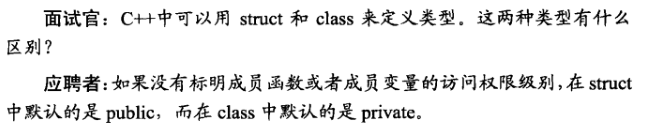面试题二  考点：实现singleton模式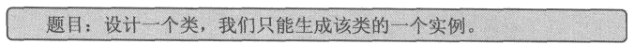（书上用c#实现的题解，一年没用c#了，我用c++写一个）

#include<iostream>

class Singleton {
Singleton() {
std::cout << "Sigletion()" << std::endl;
}
//静态成员，指向Singleton对象的指针。
static Singleton * intance;

public:
//提供静态共有方法，可以使用类名加域名进行访问，返回对象指针；
static Singleton* GetSigletion()
{
return intance;
}
};

int main()
{
Singleton *ptr = Singleton::GetSigletion();
system("pause");
return 0;
}

#include<iostream>
using namespace std;

class Singleton {
Singleton() {
cout << "Sigletion2()" << endl;
}
static Singleton* intance2;
public:
static Singleton* GetSigletion2() {
if (intance2 == NULL) {
intance2 = new Singleton();
cout << "it is once" << endl;
}else{
cout << "it is not once" << endl;
}
return intance2;
}
};

Singleton* Singleton::intance2 = NULL;  //先初始化为空，等真正用上这个单例的时候再创建这个例。

int main()
{

Singleton *ptr = Singleton::GetSigletion2();
system("pause");
return 0;
}

2.3 数据结构

2.3.1 数组

1、分析代码的运行结果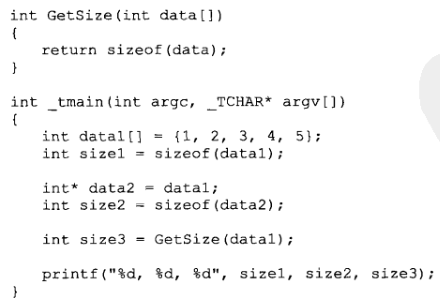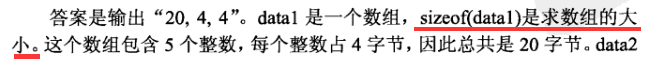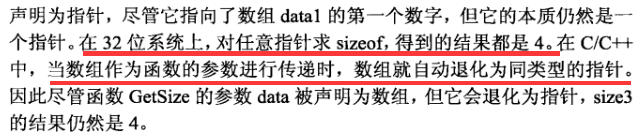面试题三   数组中重复的数字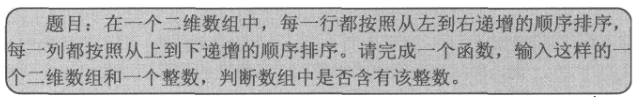class Solution {
public:
//手写快排
void QuickSort(int a[], int l,int r){
if (l >= r) return;
int low = l, high=r;
int key = a[low];
while (low < high) {
//从右往左找，如果该值小于key，则左移到low所指的位置
for (;; high--) {
if (high <= low) break;
if (a[high] < key) {
a[low] = a[high];
break;
}
}
//从左往右找，如果该值大于key，则右移到high所指的位置
for (;; low++) {
if (high <= low)break;
if (a[low] > key) {
a[high] = a[low];
break;
}
}
}
//把key放在low和high重合的位置（即key应该在数组中的最终位置）
if (low == high) a[low] = key;
//分而治之
QuickSort(a, l, low - 1);
QuickSort(a, low+1, r);
}

//用duplication指向被找到的重复数字
bool duplicate(int numbers[], int length, int* duplication) {
QuickSort(numbers, 0, length );
for(int i=0;i<length-1;i++){
if(numbers[i]==numbers[i+1]){
*duplication=numbers[i];
return true;
}
}
return false;
}
};class Solution {
public:
// Parameters:
//        numbers:     an array of integers
//        length:      the length of array numbers
//        duplication: (Output) the duplicated number in the array number
// Return value:       true if the input is valid, and there are some duplications in the array number
//                     otherwise false
bool duplicate(int numbers[], int length, int* duplication) {
if((numbers==nullptr)||length==0)  return  false;
for(int i=0;i<length;i++){
if(numbers[i]<0||numbers[i]>length-1)
return false;
}
for(int i=0;i<length;i++){
if(numbers[i]!=i){
if(numbers[numbers[i]]==numbers[i]){
* duplication=numbers[i];
return true;
}
//交换
int temp=numbers[i];
numbers[i]=numbers[temp];
numbers[temp]=temp;
}
}
return false;
}
};

/*

*/

#include "stdafx.h"
#include<iostream>
using namespace std;

int search(int start,int end,int *array,int length) {

if (array == nullptr || length <= 0) return -1;

while (end >= start)
{
int middle = (start + end) / 2;
int count = 0;
for (int i = 0; i < length; i++) {
if (array[i] >= start && array[i] <= middle) count++;
}
if (end == start) {
if (count > 1) return start;
else break;
}
if (count > (middle - start) + 1) end = middle;
else   start = middle + 1;
}
return -1;
}

int main()
{
int array = { };
int n = 7, m = 1;
for (int i = 0; i < 8; i++) {
//产生特定范围的随机数
//m <= rand() % (n - m + 1) + m <= n
array[i] = rand() % (n - m + 1) + m;
cout << array[i] << endl;
}

//数组的长度要在调用函数之前获取，因为调用函数的时候，数组退化为指针
int answer = search(1, 8, array, 8);
system("pause");
return 0;
}

面试题四  二维数组中的查找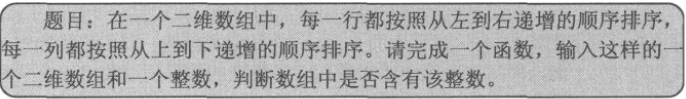#include <iostream>
#include<vector>
using namespace std;

bool Find(int target, vector<vector<int> > array) {
if (array.empty()) return false;
// array是二维数组，这里没做判空操作
int rows = array.size();
int cols = array.size();

int i = 0, j = cols - 1;
//防止数组越界，i的值等于rows时或者j的值小于0时，循环结束
//如此不用担心数组越界，因为不合法的索引无法进入循环
while (i < rows && j >= 0) {
if (target < array[i][j]) {
j--;
}
else if (target > array[i][j]) {
i++;
}
else {
return true;
}
}
return false;
}

int main()
{
vector<vector<int> >nums = { {1,2,8,9},{2,4,9,12},{4,7,10,13},{6,8,11,15}};
int target = 7;
bool answer = Find(target, nums);
return 0;
}

2.3.2 字符串

C/C++中，每个字符串都以“\0”作为结尾。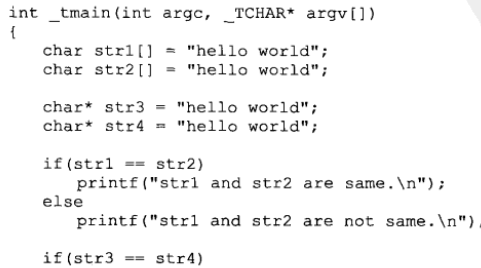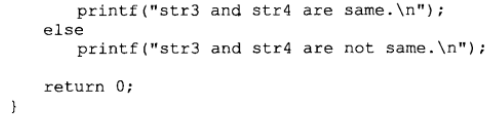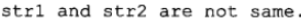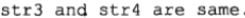面试题五 空格替换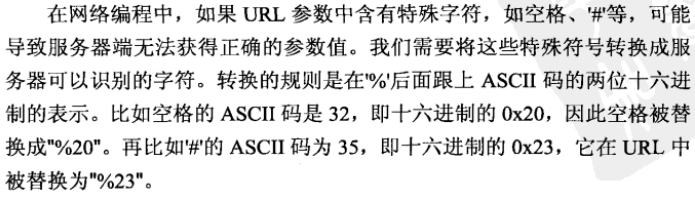class Solution {
public:
void replaceSpace(char *str,int length) {
if((str==nullptr)||(length==0)) return;
//获取数组长度的方法，记录一下
//int len=sizeof(str)/sizeof(char);
int space_num=0;
for(int i=0;i<length;i++){
if(str[i]==' ') space_num++;
}

//新字符串的长度为原字符串的长度加上两倍的空格个数
//两个长度都减一是为了防止数组越界
int new_length=length+2*space_num-1;
length-=1;

//开始替换
while(length>=0&&new_length>length){
if(str[length]==' '){
str[new_length--]='0';
str[new_length--]='2';
str[new_length--]='%';
}else{
str[new_length--]=str[length];
}
length--;
}
}
};

2.3.3 链表

struct ListNode {
int val;
ListNode * next = nullptr;
};

面试题六  从尾到头打印单链表

/**
*  struct ListNode {
*        int val;
*        struct ListNode *next;
*        ListNode(int x) :
*              val(x), next(NULL) {
*        }
*  };
*/
class Solution {
public:

}
}

//把链表头加入vector中
}
};

2.3.4 树

#include <iostream>
#include<vector>
#include<queue>

struct TreeNode {
int val;
TreeNode * left;
TreeNode * right;
TreeNode(int x)
:val(x), left(nullptr), right(nullptr){}
};

//构建二叉树
TreeNode* CreatTree(std::vector<int>&num) {
//判断边界条件
int len = num.size();
if (len == 0) return nullptr;

//数据预处理(创建索引器，索引num中的值)
int index = 0;
//创建根节点
TreeNode *root = new TreeNode(num[index]);
TreeNode * temp = nullptr;
//队列存储树的每个节点，分别为每个节点创建子节点
std::queue<TreeNode*>trans;
//根节点入队
trans.push(root);

//!trans.empty()，说明还有节点要被插入到二叉树中
while (!trans.empty() && index < len) {
temp = trans.front();
trans.pop();
//当前数组中的值非空
if (index < len && num[index] != -1) {
//构造节点
TreeNode *LeftNode = new TreeNode(num[index]);
//将构造出来的节点作为当前节点的左子树
temp->left = LeftNode;
trans.push(LeftNode);
}
index++;
if (index < len && num[index] != -1) {
TreeNode *RightNode = new TreeNode(num[index]);
temp->right = RightNode;
trans.push(RightNode);;
}
index++;
}
return root;
}

//前序遍历  根左右
void Preorder(TreeNode *root)
{
//根为空，则跳出函数
if (!root)
return;
//输出根的值
std::cout << root->val << "  ";
//递归获取左子树的值
Preorder(root->left);
//递归获取右子树的值
Preorder(root->right);
}

//中序遍历  左根右
void Midorder(TreeNode *root)
{
//根为空，则跳出函数
if (!root)
return;
//递归获取左子树的值
Midorder(root->left);
std::cout << root->val << " ";
Midorder(root->right);
}

//后序遍历  左右根
void Postorder(TreeNode *root)
{
if (!root)
return;
Postorder(root->right);
Postorder(root->left);
std::cout << root->val << "  ";
}

int main()
{
std::vector<int> num =
{ 3,5,1,6,2,0,8,-1,-1,7,4,-1,-1,-1,-1 };
TreeNode* Tree = CreatTree(num);
//前序遍历
Preorder(Tree);
//中序遍历
Midorder(Tree);
//后序遍历
Postorder(Tree);
return 0;
}

面试题七 重建二叉树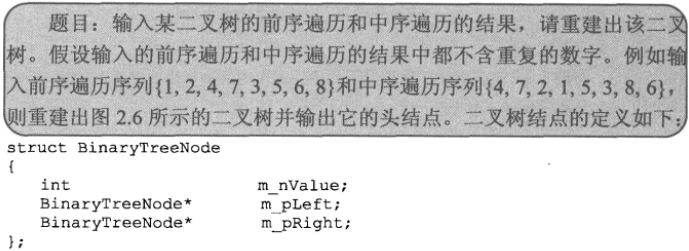class Solution {
public:
TreeNode* plantTree(vector<int> pre,vector<int> in){
//递归的退出条件
if(pre.size()==0||in.size()==0||pre.size()!=in.size())
return NULL;
TreeNode* root = new TreeNode(pre);
int index = 0;
vector<int> left_pre,right_pre,left_in,right_in;

//找到中序遍历中，根节点的位置
for(int i = 0;i<in.size();i++) {
if(root->val==in[i])
index=i;
}

//左子树的各个节点
for(int i = 0;i<index;i++) {
left_pre.push_back(pre[i+1]);
left_in.push_back(in[i]);
}

//右子树的各个节点
for(int j = index+1;j<pre.size();j++) {
right_pre.push_back(pre[j]);
right_in.push_back(in[j]);
}

root->left = plantTree(left_pre,left_in);
root->right = plantTree(right_pre,right_in);
return root;
}

TreeNode* reConstructBinaryTree(vector<int> pre,vector<int> vin) {
if(pre.empty()||vin.empty()) return nullptr;

return plantTree(pre, vin);

}
};

面试题八   二叉树的下一个节点

char转整数   -‘0’

strcpy、strstr、strlen、sizeof

+ 关注